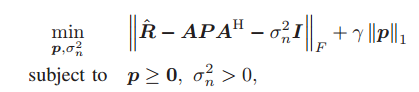# I can't solve this,who can help me?

(chenchen) #1

R is a N*N matrix.P = diag p1,p2,p3…,is a diagonal matrix of vector p.The original paper says this probem is a regularized convex optimization problem.(Mark L. Stone) #2

Is everything in the objective function, other than p, P, and \sigma^2_n, input data? If so, declare p and \sigma^2_n (not \sigma_n) to be CVX variables, and you can enter this into CVX essentially as written, using diag(p) for P.

(chenchen) #3

Thank you.i did as what you say.It is useful.And i make a transformation to this formula,then its Status: Infeasible Optimal value (cvx_optval): +Inf.everything in the objective function, other than p, P, is input data.can you teach me?

(Mark L. Stone) #4

Your second version is not equivalent to the first version. The first version should be feasible (unless the scaling is so screwed up that a solver misreports it). But th3 2nd version may or may not be feasible depending the input data - with your particular data it apparently is infeasible. If you want to solve the first problem, then enter that.

(chenchen) #5

You say the 2nd version may or may not be feasible depending the input data.Can you give me a specific example of this problem when it wil be feasible or not.The first problem was proposed and solved by others.i must make something new if want to write a paper.Thank you.I think my 2nd problem is similar to this one,right?
forgive my poor English

(Mark L. Stone) #6

Consider the trivial 1D example having R = 2, A = 0. if \sigma^2 _n < 2, your 2nd formulation is infeasible. if \sigma^2 _n \ge 2, it is feasible.

I am not in the business of doing research for you so that you can write a paper on something new. Perhaps you should spend ,more time learning the basics before trying to write a paper on something new.

This would probably not be a good research paper:
1., I took a formulation I didn’t understand.
2. I modified it to a non-equivalent formulation.
3. The non-equiavalent formulation is lousy.